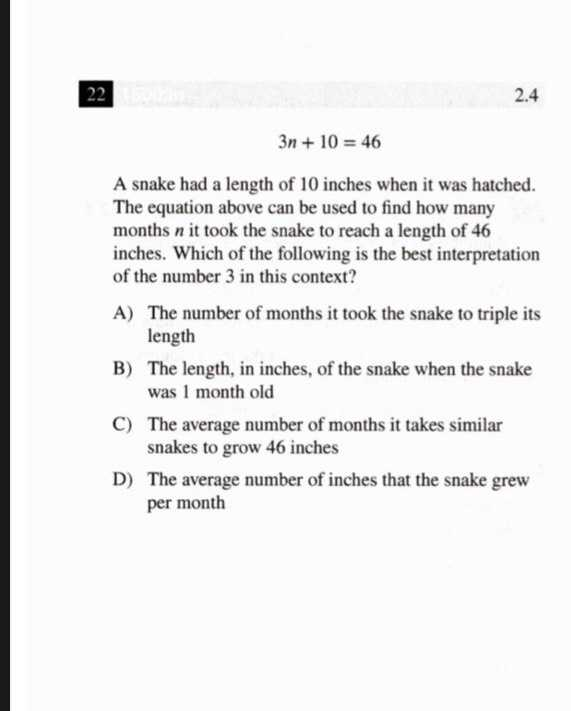### ¿Todavía tienes preguntas de matemáticas?

Pregunte a nuestros tutores expertos
Algebra
Pregunta$$3 n + 10 = 46$$

A snake had a length of $$10$$ inches when it was hatched. The equation above can be used to find how many months $$n$$ it took the snake to reach a length of $$46$$ inches. Which of the following is the best interpretation of the number $$3$$ in this context?

A) The number of months it took the snake to triple its

B) The length, in inches, of the snake when the snake

C) The average number of months it takes similar snakes to grow $$46$$ inches

D) The average number of inches that the snake grew per month